UPSC  >  Analytical Reasoning MCQ Quiz - 1

# Analytical Reasoning MCQ Quiz - 1

Test Description

## 10 Questions MCQ Test UPSC Prelims Paper 2 CSAT - Quant, Verbal & Decision Making | Analytical Reasoning MCQ Quiz - 1

Analytical Reasoning MCQ Quiz - 1 for UPSC 2023 is part of UPSC Prelims Paper 2 CSAT - Quant, Verbal & Decision Making preparation. The Analytical Reasoning MCQ Quiz - 1 questions and answers have been prepared according to the UPSC exam syllabus.The Analytical Reasoning MCQ Quiz - 1 MCQs are made for UPSC 2023 Exam. Find important definitions, questions, notes, meanings, examples, exercises, MCQs and online tests for Analytical Reasoning MCQ Quiz - 1 below.
Solutions of Analytical Reasoning MCQ Quiz - 1 questions in English are available as part of our UPSC Prelims Paper 2 CSAT - Quant, Verbal & Decision Making for UPSC & Analytical Reasoning MCQ Quiz - 1 solutions in Hindi for UPSC Prelims Paper 2 CSAT - Quant, Verbal & Decision Making course. Download more important topics, notes, lectures and mock test series for UPSC Exam by signing up for free. Attempt Analytical Reasoning MCQ Quiz - 1 | 10 questions in 10 minutes | Mock test for UPSC preparation | Free important questions MCQ to study UPSC Prelims Paper 2 CSAT - Quant, Verbal & Decision Making for UPSC Exam | Download free PDF with solutions
 1 Crore+ students have signed up on EduRev. Have you?
Analytical Reasoning MCQ Quiz - 1 - Question 1

### Find the number of triangles in the given figure.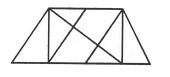Detailed Solution for Analytical Reasoning MCQ Quiz - 1 - Question 1

The figure may be labelled as shown.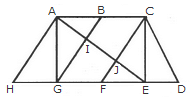The simplest triangles are AHG, AIG, AIB, JFE, CJE and CED i.e. 6 in number.

The triangles composed of two components each are ABG, CFE, ACJ and EGI i.e. 4 in number.

The triangles composed of three components each are ACE, AGE and CFD i.e. 3 in number.

There is only one triangle i.e. AHE composed of four components.

Therefore, There are 6 + 4 + 3 + 1 = 14 triangles in the given figure.

Analytical Reasoning MCQ Quiz - 1 - Question 2

### Find the number of triangles in the given figure.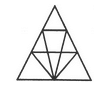Detailed Solution for Analytical Reasoning MCQ Quiz - 1 - Question 2

The figure may be labelled as shown.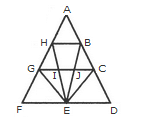The simplest triangles are AHB, GHI, BJC, GFE, GIE, IJE, CEJ and CDE i.e. 8 in number.

The triangles composed of two components each are HEG, BEC, HBE, JGE and ICE i.e. 5 in number.

The triangles composed of three components each are FHE, GCE and BED i.e. 3 in number.

There is only one triangle i.e. AGC composed of four components.

There is only one triangle i.e. AFD composed of nine components.

Thus, there are 8 + 5 + 3 + 1 + 1 = 18 triangles in the given figure.

Analytical Reasoning MCQ Quiz - 1 - Question 3

### Find the number of triangles in the given figure.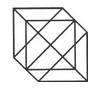Detailed Solution for Analytical Reasoning MCQ Quiz - 1 - Question 3

The figure may be labelled as shown.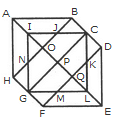The simplest triangles are IJO, BCJ, CDK, KQL, MLQ, GFM, GHN and NIO i.e. 8 in number.

The triangles composed of two components each are ABO, AHO, NIJ, IGP, ICP, DEQ, FEQ, KLM, LCP and LGP i.e.10 in number.

The triangles composed of four components each are HAB, DEF, LGI, GIC, ICL and GLC i.e. 6 in number.

Total number of triangles in the figure = 8 + 10 + 6 = 24.

Analytical Reasoning MCQ Quiz - 1 - Question 4

Find the number of triangles in the given figure.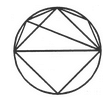Detailed Solution for Analytical Reasoning MCQ Quiz - 1 - Question 4

The figure may be labelled as shown.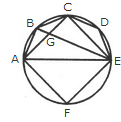The simplest triangles are ABG, BCG, CGE, CDE, AGE and AEF i.e. 6 in number.

The triangles composed of two components each are ABE, ABC, BCE and ACE i.e. 4 in number.

There are 6 + 4 = 10 triangles in the figure.

Analytical Reasoning MCQ Quiz - 1 - Question 5

Find the number of triangles in the given figure.Detailed Solution for Analytical Reasoning MCQ Quiz - 1 - Question 5

The figure may be labelled as shown.The simplest triangles are BFG, CGH, EFM, FMG, GMN, GHN, HNI, LMK, MNK and KNJ i.e. 10 in number.

The triangles composed of three components each are FAK and HKD i.e. 2 in number.

The triangles composed of four components each are BEN, CMI, GLJ and FHK i.e. 4 in number.

The triangles composed of eight components each are BAJ and OLD i.e. 2 in number.

Thus, there are 10 + 2 + 4 + 2 = 18 triangles in the given figure.

Analytical Reasoning MCQ Quiz - 1 - Question 6

Count the number of rectangles in the given figure.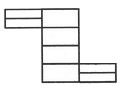Detailed Solution for Analytical Reasoning MCQ Quiz - 1 - Question 6

The figure may be labelled as shown.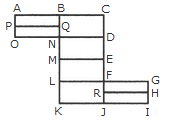The simplest rectangles are ABQP, PQNO, BCDN, NDEM, MEFL, LFJK, FGHR and RHIJ i.e. 8 in number.

The rectangles composed of two components each are ABNO, BCEM, NDFL, MEJK and FGIJ i.e. 5 in number.

The rectangles composed of three components each are ACDO, BCFL, NDJK and LGIK i.e. 4 in number.

There is only one rectangle i.e. BCJK composed of four components.

Total number of rectangles in the figure = 8 + 5 + 4 + 1 = 18.

Analytical Reasoning MCQ Quiz - 1 - Question 7

How many circles are there in the adjoining figure.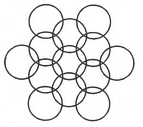Detailed Solution for Analytical Reasoning MCQ Quiz - 1 - Question 7

The figure may be labelled as shown.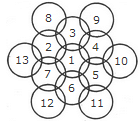There are 13 circles in the given figure. This is clear from the adjoining figure in which the centres of all the circles in the given figure have been numbered from 1 to 13.

Analytical Reasoning MCQ Quiz - 1 - Question 8

What is the minimum number of straight lines that is needed to construct the figure?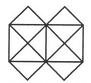Detailed Solution for Analytical Reasoning MCQ Quiz - 1 - Question 8

The figure may be labelled as shown.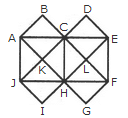The horizontal lines are AE and JF i.e. 2 in number. The vertical lines are AJ, CH and EF i.e. 3 in number.

The slanting lines are AG, BF, JD, IE, AB, DE, JI and FG i.e. 8 in number.

Total number of straight lines needed to construct the figure = 2 + 3 + 8 = 13.

Analytical Reasoning MCQ Quiz - 1 - Question 9

Determine the number of rectangles and hexagons in the given figure.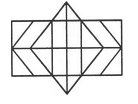Detailed Solution for Analytical Reasoning MCQ Quiz - 1 - Question 9

The figure may be labelled as shown.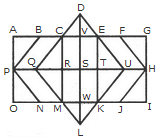Rectangles:

The simplest rectangles are CVSR, VETS, RSWM and STKW i.e. 4 in number.

The rectangles composed of two components each are CETR, VEKW, RTKM and CVWM i.e. 4 in number.

The rectangles composed of three components each are ACRP, PRMO, EGHT and THIK i.e. 4 in number.

The rectangles composed of four components each are CEKM, AVSP,PSWO, VGHS and SHIW i.e. 5 in number.

The rectangles composed of five components each are AETP, PTKO, CGHR and RHIM i.e. 4 in number.

The rectangles composed of six components each are ACMO and EGIK i.e. 2 in number.

The rectangles composed of eight components each are AGHP, PHIO, AVWO and VGIW i.e. 4 in number.

The rectangles composed of ten components each are AEKO and CGIM i.e. 2 in number.

AGIO is the only rectangle having sixteen components.

Total number of rectangles in the given figure

= 4 + 4 + 4 + 5 + 4 + 2 + 4 + 2 + 1 = 30.

Hexagons:

The hexagons in the given figure are CDEKLM, CEUKMQ, CFHJMQ, BEUKNP and BFHJNP.

So, there are 5 hexagons in the given figure.

Analytical Reasoning MCQ Quiz - 1 - Question 10

Count the number of triangles and squares in the given figure.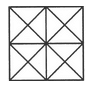Detailed Solution for Analytical Reasoning MCQ Quiz - 1 - Question 10

The figure may be labelled as shown.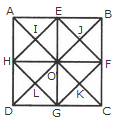Triangles :

The simplest triangles are AEI, EOI, OHI, HAI, EBJ, BFJ, FOJ, OEJ, HOL, OGL, GDL, DHL, OFK, FCK, CGK and GOK i.e. 16 in number.

The triangles composed of two components each are HAE, AEO, EOH, OHA, OEB, EBF, BFO, FOE, DHO, HOG, OGD, GDH, GOF, OFC, FCG and CGO i.e. 16 in number.

The triangles composed of four components each are HEF, EFG, FGH, GHE, ABO, BGO, CDO and DAO i.e. 8 in number.

The triangles composed of eight components each are DAB, ABC, BCD and CDA i.e. 4 in number.

Total number of triangles in the figure = 16 + 16 + 8 + 4 = 44.

Squares :

The squares composed of two components are HIOL, IEJO, JFKO and KGLO i.e. 4 in number.

The squares composed of four components are AEOH, EBFO, OFGC and HOGD i.e.4 in number.

There is only one square EFGH which is composed of eight components.

There is only one square ABCD which is composed of sixteen components.

Total number of squares in the figure = 4 + 4 + 1 + 1 = 10.

## UPSC Prelims Paper 2 CSAT - Quant, Verbal & Decision Making

67 videos|50 docs|151 tests
 Use Code STAYHOME200 and get INR 200 additional OFF Use Coupon Code
Information about Analytical Reasoning MCQ Quiz - 1 Page
In this test you can find the Exam questions for Analytical Reasoning MCQ Quiz - 1 solved & explained in the simplest way possible. Besides giving Questions and answers for Analytical Reasoning MCQ Quiz - 1, EduRev gives you an ample number of Online tests for practice

## UPSC Prelims Paper 2 CSAT - Quant, Verbal & Decision Making

67 videos|50 docs|151 tests

### How to Prepare for UPSC

Read our guide to prepare for UPSC which is created by Toppers & the best Teachers